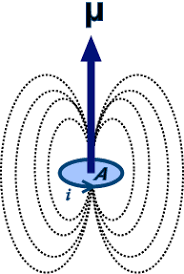## How to Calculate and Solve for Magnetic Moment of a Dipole | Magnetic FieldThe image above represents magnetic moment of a diople.

To compute for magnetic moment of a diople, two essential parameters are needed and these parameters are Magnetic Pole Strength (m) and Distance Seperating Poles (l).

The formula for calculating magnetic moment of a diople:

M = ml

Where;

M = Magnetic Moment of a Diople
m = Magnetic Pole Strength
l = Distance Seperating Poles

Let’s solve an example;
Find the magnetic moment of a diople when the magentic pole strength is 21 and the distance seperating poles is 13.

This implies that;

m = Magnetic Pole Strength = 21
l = Distance Seperating Poles = 13

M = ml
M = 21 x 13
M = 273

Therefore, the magnetic moment of a diople is 273.

Calculating the Magnetic Pole Strength when the Magnetic Moment of a Diople and the Distance Seperating Poles is Given.

m = M / l

Where;

m = Magnetic Pole Strength
M = Magnetic Moment of a Diople
l = Distance Seperating Poles

Let’s solve an example;
Find the magnetic pole strength when the magnetic moment of a diople is 30 and the distance seperating poles is 5.

This implies that;

M = Magnetic Moment of a Diople = 30
l = Distance Seperating Poles = 5

m = M / l
m = 30 / 5
m = 6

Therefore, the magnetic pole strength is 6.